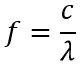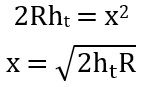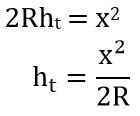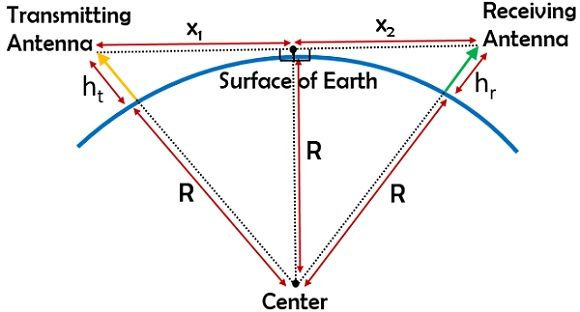# Space Wave Propagation

Definition: Space wave propagation is the type of radio wave propagation in which the radio waves are propagated either directly from transmitting antenna to receiving antenna or by getting reflected from the ground. Basically in space wave propagation, direct transmission of the signal is achieved by line of sight communication.

The transmission of a signal between transmitter and receiver is achieved in the tropospheric region of the atmosphere. Thus space wave propagation is sometimes referred as tropospheric wave propagation. This type of radio wave propagation allows the transmission of signals having a very large range of frequencies. Usually, it permits the transmission of signals having a frequency greater than 30 MHz.

## Content: SpaceWave Propagation

### Need for space wave propagation?

Basically, space wave propagation has been adopted to overcome the disadvantages associated with ground wave propagation and sky wave propagation.

We have already discussed that ground wave propagation leads to cause attenuation of signal due to neutralization. Thus the frequency of the signal to be transmitted is kept low. Also, not to have much signal attenuation, the propagation distance is kept small. However, sky wave propagation permits the transmission of signals with comparatively greater frequency than ground wave propagation with less attenuation. But even sky wave propagation fails when there exists a need for transmitting extremely high-frequency signals. This is so because we know in sky wave propagation the signals get transmitted by being reflected from the ionosphere.

But beyond that particular range of frequency, despite undergoing reflection, the signal penetrates the atmosphere. Thus is lost without getting received at the destination. So, space wave propagation is used for propagating high-frequency signals by permitting direct communication between the transmitting and receiving antenna. In the case of space wave propagation, the signal without being reflected from the ionosphere simply gets propagated from an end to another in the line of sight range.

As we know that line of sight (LOS) communication is achieved between 2 points that are free from obstruction. Now the question arises why such a high-frequency signal transmission requires LOS communication?

So, we know the basic relationship between f and λ is given as:As frequency and wavelength are inversely proportional to each other. Thus higher the frequency, the smaller will be the wavelength. And an electromagnetic wave of a small wavelength cannot easily penetrate the obstacle present in the path. Thus due to the range of frequency involved in space wave propagation, LOS communication is necessary.

It is to be noted here that the range of signal propagation from the troposphere is limited by the curvature of the earth.

## What is Space Wave Propagation?

In space wave propagation, the electromagnetic waves are propagated when a direct path of communication is present between the sender and receiver. Sometimes the waves are transmitted by getting reflected from the ground.

The radio waves with extremely high frequencies are known as space waves. The troposphere allows the propagation of such waves. Generally, the troposphere is extended up to 10 to 20 km above the surface of the earth. Thus space wave propagation occurs at about 20 km region in the atmospheric zone. Sometimes called the line of sight communication. This is so because such high-frequency waves require a direct path for propagation.

The figure below represents the transmission of space waves:As we can see in the figure shown above that the transmitting and receiving antennas are placed in a range where the two are present at a visible distance with respect to each other. So, in this case, the signal emitted by the transmitting antenna gets directly received by the receiving antenna in the absence of any obstacle in the region present in between the two antennas.

This is so because, for extremely small wavelengths, the signal will suffer attenuation in the presence of an obstruction in the path of propagation.

Now another major point of concern in the case of space wave propagation is, how the range of signal transmission is specified and how can we detect the range up to which the two must be separated while installation on the surface of the earth. So, the range of signal transmission is related to the height of the antennas concerned.

To have a proper line of sight propagation above the surface of the earth, the orientation of the antennas must be such that the propagated wave must not interact with the curvature of the earth. However, for extremely low height antennas, the waves get reflected by the ground under certain conditions. And the reflected wave is received by the antenna at the other end. So, to have direct LOS communication between the two antennas, the antenna units must be placed with proper orientation on the ground.

### Range Detection in Space Wave Propagation

Let us first consider a single transmitting antenna having height ht, propagating the signal up to a certain range on the ground.Suppose x specifies the range between the transmitting antennas and the center of the earth at the curvature where the signal is getting received and R is the radius of curvature of the earth.

Now, we will apply Pythagoras theorem in the above triangle, soOn simplifying further,As the height of the antenna is less in comparison to the radius of the earth. So ‘ht2’ can be neglected from the above equation.

So, on further simplification, we can have,So, we can say that the range of signal transmission shows dependency on the height of the antenna which is transmitting the signal.

Thus it can be concluded that if the range of propagation is known then one can determine the respective height of the transmitting antenna, by using the relationship:Suppose the transmitted signal must be received by another antenna on the ground. So, the question arises what must be the height of the receiving antenna in order to have LOS communication.

So, consider the figure below:Here two antennas are placed on the surface of the earth. Let the height of transmitting and receiving antennas be ht and hr respectively. Also, the range from a common point on the curvature to transmitting and receiving antenna is xt and xr. Then individually we will have:So, on combining the above two equations we will have the permissible range between two antennas for LOS communication. Therefore,Hence, this expression provides the range that allows LOS communication for the two respective heights of the antennas.

In the case of space wave propagation for an extremely large range of signal propagation, multiple antennas must be placed in the geometry at specific distances in a way that, each antenna will permit LOS communication with its adjacent antennas.

We know that the general formula for the area is given as πr2. Since here the range is 2hR. Hence the region in terms of area which is covered by transmitting antenna is given as:Neglecting π we get,As we can see that the area and height of the antenna are directly proportional to each other. Thus higher the height of the antenna, the greater will be the area covered by it.

### Applications

As, this type of propagation, permits large frequency operation, thus finds applications in satellite and radar communication, etc. along with microwave linking applications.